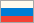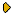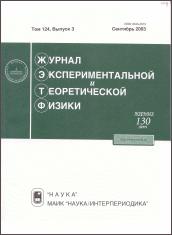Journal of Experimental and Theoretical Physics
 HOME | SEARCH | AUTHORS | HELPJournal IssuesGolden PagesAbout This journalAims and ScopeEditorial BoardManuscript SubmissionGuidelines for AuthorsManuscript StatusContactsDear users! "JETP" web site has moved to new address: http://jetp.ras.ru. Please update your bookmarks! Latest issue ZhETF, Vol. 162, No. 4, October 2022 ZhETF - Current volume 162 No 4, October 2022No 3, September 2022No 2, August 2022No 1, July 2022 Russian version archive (ZhETF) Vol. 162, 2022Vol. 161, 2022Vol. 160, 2021Vol. 159, 2021Vol. 158, 2020Vol. 157, 2020Vol. 156, 2019Vol. 155, 2019Vol. 154, 2018Vol. 153, 2018Vol. 152, 2017Vol. 151, 2017Vol. 150, 2016Vol. 149, 2016Vol. 148, 2015Vol. 147, 2015Vol. 146, 2014Vol. 145, 2014Vol. 144, 2013Vol. 143, 2013Vol. 142, 2012Vol. 141, 2012Vol. 140, 2011Vol. 139, 2011Vol. 138, 2010Vol. 137, 2010Vol. 136, 2009Vol. 135, 2009Vol. 134, 2008Vol. 133, 2008Vol. 132, 2007Vol. 131, 2007Vol. 130, 2006Vol. 129, 2006Vol. 128, 2005Vol. 127, 2005Vol. 126, 2004Vol. 125, 2004Vol. 124, 2003Vol. 123, 2003Vol. 122, 2002Vol. 121, 2002Vol. 120, 2001Vol. 119, 2001Vol. 118, 2000Vol. 117, 2000Vol. 116, 1999Vol. 115, 1999Vol. 114, 1998Vol. 113, 1998Vol. 112, 1997Vol. 111, 1997Vol. 110, 1996Vol. 109, 1996Vol. 108, 1995Vol. 107, 1995Vol. 106, 1994Vol. 105, 1994Vol. 104, 1993Vol. 103, 1993Vol. 102, 1992Vol. 101, 1992Vol. 100, 1991Vol. 99, 1991Vol. 98, 1990Vol. 97, 1990Vol. 96, 1989Vol. 95, 1989Vol. 94, 1988Vol. 93, 1987Vol. 92, 1987Vol. 91, 1986Vol. 90, 1986Vol. 89, 1985Vol. 88, 1985Vol. 87, 1984Vol. 86, 1984Vol. 85, 1983Vol. 84, 1983Vol. 83, 1982Vol. 82, 1982Vol. 81, 1981Vol. 80, 1981Vol. 79, 1980Vol. 78, 1980Vol. 77, 1979Vol. 76, 1979Vol. 75, 1978Vol. 74, 1978Vol. 73, 1977Vol. 72, 1977Vol. 71, 1976Vol. 70, 1976Vol. 69, 1975Vol. 68, 1975Vol. 67, 1975Vol. 66, 1974Vol. 65, 1974Vol. 64, 1973Vol. 63, 1973Vol. 62, 1972Vol. 61, 1972Vol. 60, 1971Vol. 59, 1971Vol. 58, 1970Vol. 57, 1970Vol. 56, 1969Vol. 55, 1969Vol. 54, 1968Vol. 53, 1968Vol. 52, 1967Vol. 51, 1967Vol. 50, 1966Vol. 49, 1966Vol. 48, 1965Vol. 47, 1965Vol. 46, 1964Vol. 45, 1964Vol. 44, 1963Vol. 43, 1963Vol. 42, 1962Vol. 41, 1962Vol. 40, 1961Vol. 39, 1961Vol. 38, 1960Vol. 37, 1960Vol. 36, 1959Vol. 35, 1959Vol. 34, 1958Vol. 33, 1958Vol. 32, 1957Vol. 31, 1957Vol. 30, 1956Vol. 29, 1956Vol. 28, 1955 English version archive (JETP, 1967-1996) Vol. 83, 1996Vol. 82, 1996Vol. 81, 1995Vol. 80, 1995Vol. 79, 1994Vol. 78, 1994Vol. 77, 1993Vol. 76, 1993Vol. 75, 1992Vol. 74, 1992Vol. 73, 1991Vol. 72, 1991Vol. 71, 1990Vol. 70, 1990Vol. 69, 1989Vol. 68, 1989Vol. 67, 1988Vol. 66, 1987Vol. 65, 1987Vol. 64, 1986Vol. 63, 1986Vol. 62, 1985Vol. 61, 1985Vol. 60, 1984Vol. 59, 1984Vol. 58, 1983Vol. 57, 1983Vol. 56, 1982Vol. 55, 1982Vol. 54, 1981Vol. 53, 1981Vol. 52, 1980Vol. 51, 1980Vol. 50, 1979Vol. 49, 1979Vol. 48, 1978Vol. 47, 1978Vol. 46, 1977Vol. 45, 1977Vol. 44, 1976Vol. 43, 1976Vol. 42, 1975Vol. 41, 1975Vol. 40, 1975Vol. 39, 1974Vol. 38, 1974Vol. 37, 1973Vol. 36, 1973Vol. 35, 1972Vol. 34, 1972Vol. 33, 1971Vol. 32, 1971Vol. 31, 1970Vol. 30, 1970Vol. 29, 1969Vol. 28, 1969Vol. 27, 1968Vol. 26, 1968Vol. 25, 1967Vol. 24, 1967Vol. 23, 1966Vol. 22, 1966Vol. 21, 1965Vol. 20, 1965Vol. 19, 1964Vol. 18, 1964Vol. 17, 1963Vol. 16, 1963Vol. 15, 1962Vol. 14, 1962Vol. 13, 1961Vol. 12, 1961Vol. 11, 1960Vol. 10, 1960Vol. 9, 1959Vol. 8, 1959Vol. 7, 1958Vol. 6, 1958Vol. 5, 1957Vol. 4, 1957Vol. 3, 1956Vol. 2, 1956Vol. 1, 1955 Accepted manuscripts Dear authors! In accordance with MAIK regulations, from September 1, 2016 manuscripts submitted in English will be translated in Russian for the Russian version of our journal.Print ISSN: 0044-4510
Report problems